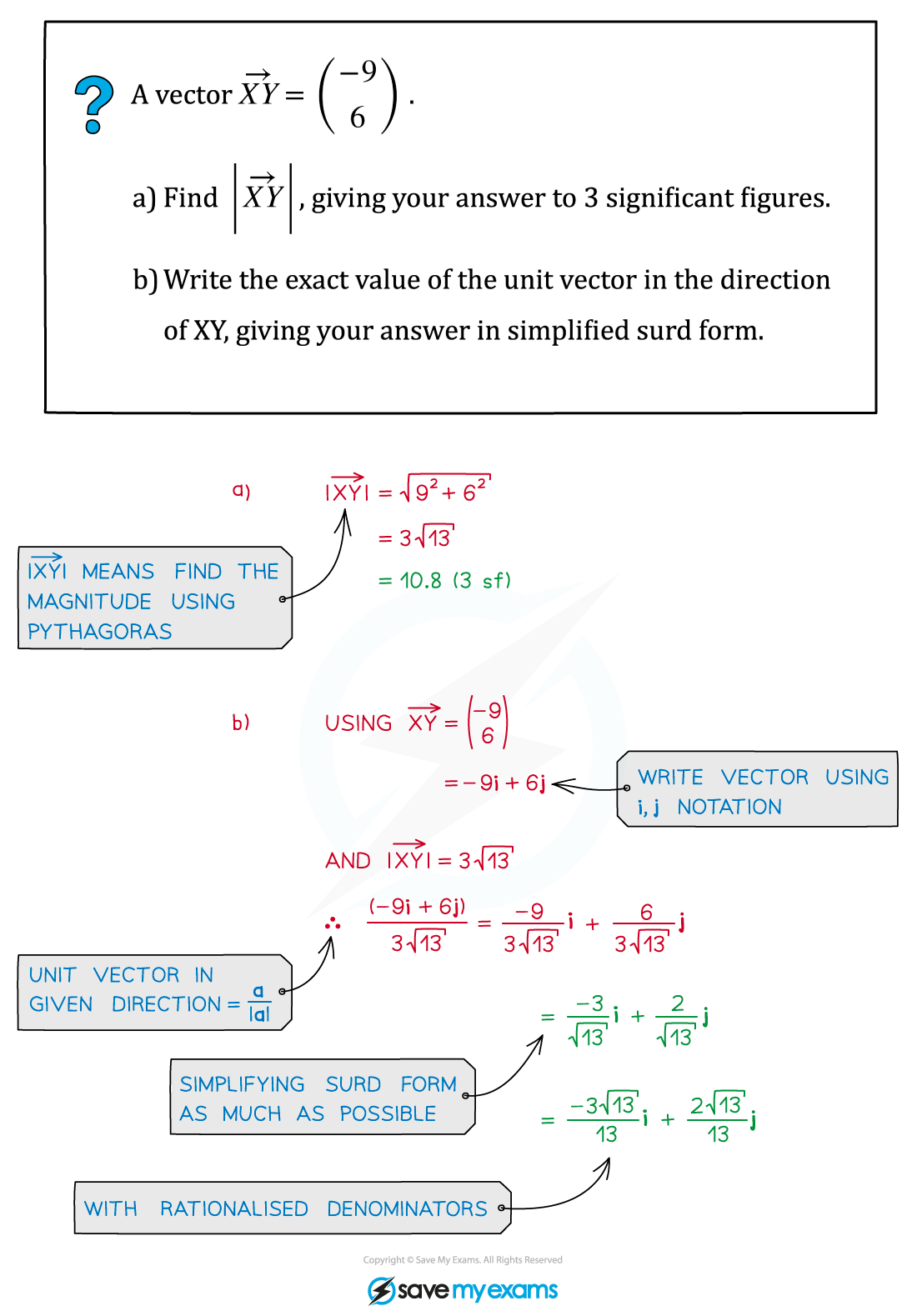# CIE A Level Maths: Pure 3复习笔记7.1.2 Magnitude & Direction

### Magnitude & Direction

#### What is the magnitude of a vector?

• The magnitude of a vector is simply its size
• It also tells us the distance between two points
• You can find the magnitude of a vector using Pythagoras’ theorem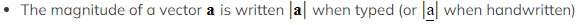• To work out the unit vector in the direction of a given vector
• unit vector has a magnitude of 1So to find the unit vector of a given vector, divide by its magnitude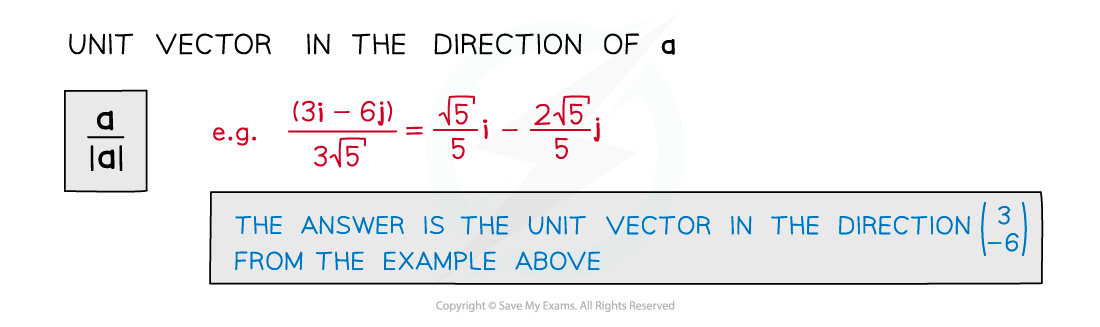#### What is the direction of a vector?

• Vectors have opposite direction if they are the same size but opposite signs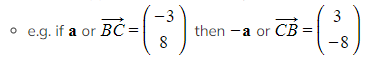• The direction of a vector is what makes it more than just a scalar
• Eg. two objects with velocities of 7 m/s and ‑7 m/s are travelling at the same speed but in opposite directions
• Two vectors are parallel if and only if one is a scalar multiple of the other
• For real-life contexts such as mechanics, direction can be calculated from a given vector using trigonometry (see Right-Angled Triangles)
• It is usually calculated anticlockwise from the positive x-axis (unless otherwise stated eg. a bearing)

#### How do I write a vector in component form?

• We have already seen that vectors can be written in different forms
• Component form means writing a vector in terms of i and j components
• Given the magnitude and direction of a vector you can work out its components and vice versa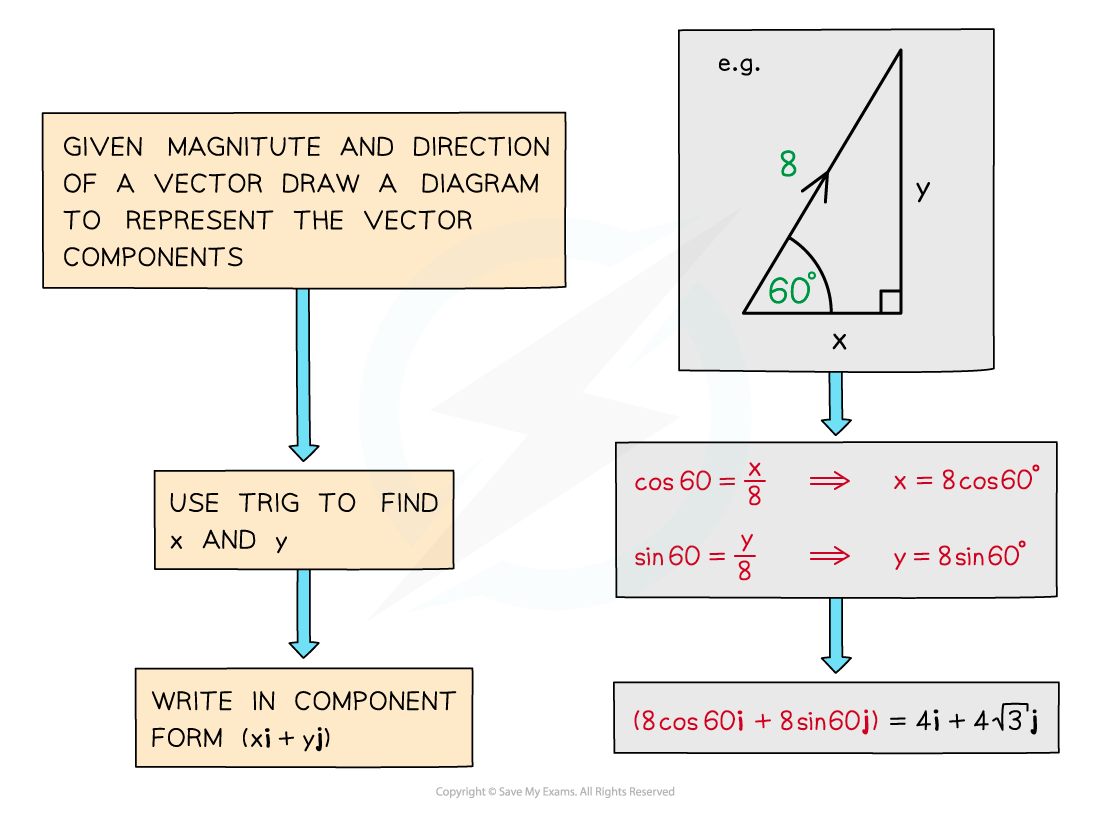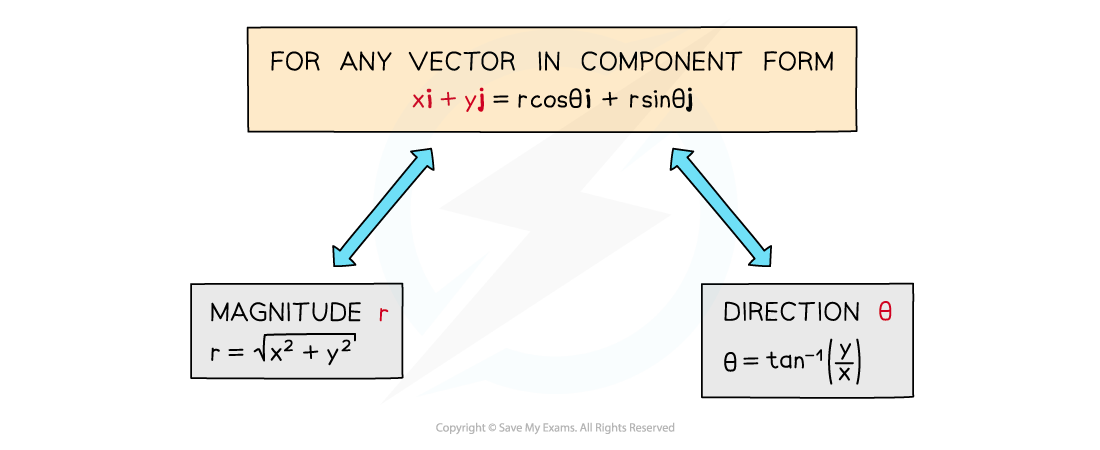#### Exam Tip

• Diagrams can help, especially when working out direction – if there isn’t a diagram, draw one.
• Remember, resolving a vector just means writing it in component form.

#### Worked Example